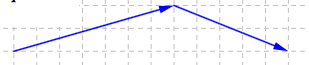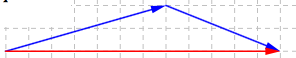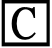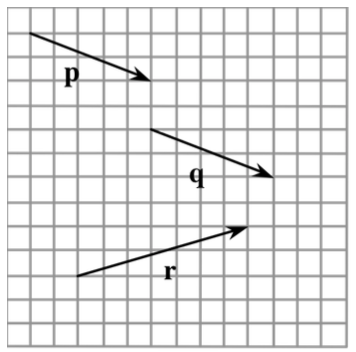### Home > PC > Chapter 10 > Lesson 10.1.1 > Problem10-10

10-10.

Copy the vector diagram on graph paper.

1. Draw vectors equivalent to r and q so that you can add them easily.2. Draw and label the resultant vector, r + q.3. Using the same initial point, draw and label q + r.

Move vectors r and q above to show q + r.

4. The quadrilateral you just drew has a special name. What is it?

5. Challenge: You can prove that r + q = q + r by proving that your answer to part (d) is true. Use what you remember from geometry to justify the special name.